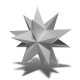# Math factoid of the day: 56

56 is the sum of six consecutive primes (3 + 5 + 7 + 11 + 13 + 17), and is a pronic number, being the product of two consecutive numbers (7 × 8).

» 2 reactionsLet's get some things straight: A prionic number is a number with mad cow disease. A priapic number is 1. A pronic number is 7x8.Thanks, fixed :)